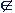## Sets

1. Definition of a set

A set is a collection of well defined objects.

Examples:

{1, 3, 5, 7, 9} is a set of positive odd numbers less than 10.

The property that the numbers 1, 3, 5, 7 and 9 are odd is their being well defined.

Africa, Asia, America, Australia, Europe are sets of continents in this world.

All students in a class whose names begin with a consonant.

Continent is the well defined property shared by the lands of the above names.

Note:

1. A set is denoted by braces {} within which the members of the set are written.

2. The capital letters are used for denoting sets and small letters for members of the sets.

2. Members/Elements of a set

The members of a set are called its elements.

In the set {1, 3, 5, 7, 9}, the numbers 1, 3, 5, 7, 9 are called members of the set or more precisely elements of the set {1, 3, 5, 7, 9}

The symbol ‘’ denotes ‘belongs to’ or it stands for ‘element of’.

Therefore we write 1 {1, 3, 5, 7, 9}, which is read as

‘1 is an element of (or belongs to) the set {1, 3, 5, 7, 9}. Likewise other elements of the set can be similarly denoted.

The symbol ‘’ denotes “does not belong to” or ‘is not an element of’

So, we can translate 2{1, 3, 5, 7, 9} as

2 is not an element of the set {1, 3, 5, 7, 9}, or

2 does not belong to the set {1, 3, 5, 7, 9}.

Note:

Let A = {1, 3, 5, 7, 9} and B = {5, 7, 9, 1, 3}.

Now are the two sets A and B different?

No. Why?

Because a set is same irrespective of the order in which the elements are written.

3. Denotation of a Set/Representation of a set.

There are three ways of denoting a set.

Descriptive method

In this method, the set is described in complete words in a sentence.

Example 1:

The set A = {1, 2, 3, 4, 5... 100} can be described as

“A is a set of all the consecutive integers from 1 to 100”.

Note:

The three periods after 5 upto 100 stand for all the integers between the two numbers.

Roster method

In this method, the set is represented by writing its elements inside braces

{ }

Example 1:

A = {3, 6, 9, 12, 15}

A is a set of the first five positive multiples of 3.

X = {a, e, i, o, u}

X is a set of the vowels in the English alphabets.

Set builder form

In this method of representation, the set is described using the unique property shared by all of the elements of the set.

Consider the set

Example 1:

A = {2, 3, 5, 7}

What is the common property shared by the elements 2, 3, 5 and 7.

They are all prime numbers less than 10.

In set builder form, also called Rule method, we write it as

A = {x/x is a prime number less than 10} expanded as

“A is a set of elements x, such that x is a prime number less than 10”

Example 2:

Consider in Roster form the following set

X = {3, 6, 9, 12, 15, 18}.

In set builder form, it is

X = {x/x = 3n, where n ∈N and n < 5}

n ∈N stands for ‘n belongs to the set of natural numbers less than 5

the formula for set builder form is therefore,

S = {x/x has a property} or S = {x: x has a property}